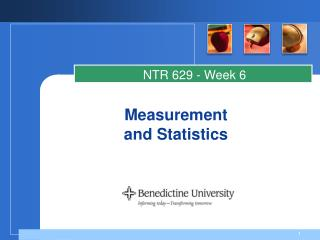DownloadDownload PresentationMeasurement and Statistics

# Measurement and Statistics

Download Presentation## Measurement and Statistics

- - - - - - - - - - - - - - - - - - - - - - - - - - - E N D - - - - - - - - - - - - - - - - - - - - - - - - - - -
##### Presentation Transcript

1. NTR 629 - Week 6 Measurementand Statistics

2. What is Measurement • Measurement is the assignment of values to outcomes • Numerical examples: • 80% accuracy • Categorical examples: • High (vs. low)

3. 4 Levels of Measurement • Nominal • Group (categorize) and label data • Examples: gender; grade; number on sports jersey • Ordinal • Rank (greater, equal or less than; high or low) variables across a continuum. Intervals between not interpretable. • Example: position in a race. • Interval • Equal differences between scores • Examples: temperature on thermometer; Likert scale • Ratio • Variables with equal intervals between and absolute zero (meaning absence of variable); twice as much has meaning • Example: test scores

4. Statistics Overview • Descriptive • Distribution characteristics of a set of data • Inferential • Test the significance • Null hypothesis •  < .05 or provide exact  value

5. Descriptive Statistics • Distribution of scores • Frequency distribution • Range (difference between highest and lowest) • Measures of central tendency • Mean (interval and ratio data) • Median (ordinal data) • Mode (nominal data) • Measures of variability • Standard deviation

6. Inferential Statistics Experimental Research • What is your research exploring? • Differences? • Number (or level) of variables? • Decision Tree • Parametric • e.g., t-test, ANOVA • Nonparametric • e.g., Chi-square, Mann Whitney, Wilcoxon, Wilcox, Kruskal-Willis Nonexperimental Research • What is your research exploring? • Relationships (Correlation Research)? • Differences in Ranks? • Decision Tree • Parametric correlation • Pearson r • Nonparametric correlation • Spearman rho • Nonparametric ranking • Friedman

7. Selecting a Statistical Test • Look at research questions/hypothesis and determine the type of statistical test: • What type of data (i.e., measurement type)? • Nature of question/hypothesis? • Are you describing or comparing? • Are you asking about relationships? • Are you comparing test scores? • Are you comparing levels of achievement? • Are you examining the distribution of scores or the association of scores to gender and age (groups)? • How many independent variables? • How many dependent variables? • http://www.ats.ucla.edu/stat/mult_pkg/whatstat/default.htm (choosing a statistical test)